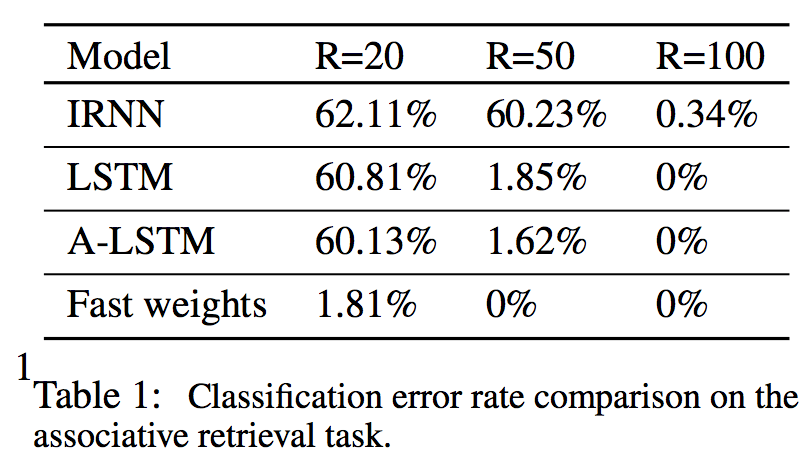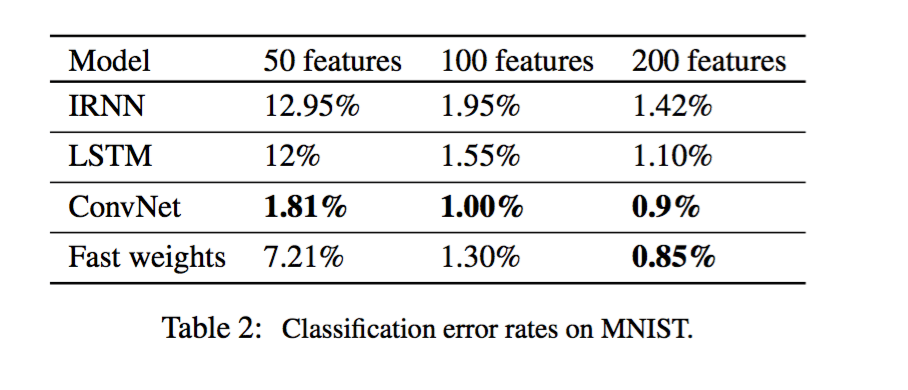# Geoffrey Hinton: Using Fast Weights to Store Temporary Memories

Machine learning Advances and Applications Seminar address how to use fast weights to effectively store temporary memories, at University of Toronto

Toronto, Canada, University of Toronto Fields Institute, Machine Learning Advances and Applications Seminar Series  October 13, 2016

This lecture talked about the fast weights idea and its realization. “Fast weights” can be used to store temporary memories of the recent past, and they provide a neurally plausible way of implementing the type of attention to the past that has recently proved very helpful in sequence-to-sequence models. By using fast weights, we can avoid the need to store copies of neural activity patterns.

The basic idea here is that temporary memories can be stored not by adding active neurons, but by having some changes to the existing neurons.

For each neuron connection, the weight that is associated with the connection has two components. One is the standard slow weight, which represents long term memory – learning slowly and decay slowly. The other one is the fast weight, which represents short term memory – learning quickly and decay quickly. The fast weight exist in – for example – priming: you hear a word with lots of noise. If this is the first time you heard this word, you may not recognize it. But if you heard this word 30 minutes prior, you are able to recognize it because the threshold of being able to recognize this word is less than 30 minutes’ memory.

Generally speaking, imagine a localist representation: in order to achieve the above effect, we can just temporarily lower the threshold of neuron for that specific word instead of changing the whole structure. The other way to think about it is imagine having a pattern of activities: you can change the weight of the neuron between pattern of activities, to make a specific activity become a stronger attractor (ex. easier to recognize a word). This is how we store memory: we change weights to store that pattern instead of storing the pattern. Because, if we store short term memory with weights matrix instead of activity vector, we can get a lot more capacity with limited neurons.

There are two way to store temporary knowledge:

1. LSTM: Store activities in hidden unit, has very limited capacity. Assume the hidden state has H units, LSTM is limited to short term memory (attractor) of O(H) for the history of the current sequence (from paper). In addition, LSTM stores information in its hidden unit, such that the short term memory is irrelevant to the ongoing process.
2. Fast weights: Allows you to store short term memory with weights matrix, resulting in higher capacity. In addition, the short term memory could store information specific to the history of the current sequence, such that the information is available to affect the ongoing process.

How can we apply the fast weights idea?

Let’s start with ReLU RNNs. ReLU RNNs are easy to train and good at learning long term dependency (long term memory). The picture on the left shows the simple RNN, the right is an identical RNN if we initialize the hidden “→” : hidden weights shown by the blue arrow with the null matrix. In order to apply fast weights idea, the outer product learning rule has been used here to update fast weights matrix A.Updating rule of the fast weights matrix A: Every time we learned a hidden state h(t) at time step t, we multiply the current fast weights A(t-1) by the weight decay parameter λ, and add the outer product of the newly learned hidden state h(t), multiplying by learning rate η. The full updating function is shown below. The fast weights matrix would be the sum of the contribution from the past and the contribution declined over time. The contribution is the outer product h(t)h(t)^T of the hidden state.

A(t) = λA(t − 1) + ηh(t)h(t)^T
hs+1(t + 1) = f([Wh(t) + Cx(t)] + A(t)hs(t + 1))Adding layer normalization (LN) tricks make it work better

In a standard RNN, there is a tendency for the average magnitude of the summed inputs for the recurrent units to either grow or shrink at every time-step, leading to exploding or vanishing gradients. LN is saying that before apply non-linearity(ex. ReLU) to the vector of total input received by the hidden unit, normalized the vector to zero mean and unit variance first. In a layer normalized RNN, the normalization terms make it invariant to re-scaling all of the summed inputs to a layer, which results in a much more stable hidden-to-hidden dynamics.

Does it work?

Let’s demonstrate the effectiveness using two tasks:

Task: Consider a task where multiple key-value pairs are present in a sequence as input. Convert the input sequence into a R dimension vector, which serves as an input to the recurrent hidden unit, and check the error rate of this task

Results: The result table below shows that a RNN can solve this problem with a 100 dim hidden unit, a LSTM can solve with a 50 dim hidden unit, and the fast weights RNN only need a 20 dim hidden unit. This is unsurprising, because 20 dim hidden unit in FW RNN has a much larger capacity than in a regular RNN. The question then becomes: can it learn how to use that much memory capacity? The answer: yes, because FW RNN got an error rate of 1.18% for R=20 setting.Task two – Using Fast Weights to combine glimpses

Background: Visual attention models have been shown to overcome some limitation of ConvNet. One of them is understanding where the ConvNet is looking at. Another is that attention model is able to selectively focus on important parts in the image.

How does attention models work? Given an input image, a visual attention model computes a set of glimpses. Each glimpse corresponds to a small region of the image. Visual attention model can learn to find multiple objects in the image and classify them correctly, but the way it uses to compute glimpses is over-simplistic: it uses a single scale of glimpses and scan over the whole image with specific order. However, human eyes are capable of focusing on different part of the image with different scale and integrate them together to make the right decision. Improving the model’s ability to remember recent glimpses should help the visual attention model discover non-trivial glimpse policies. Fast weights can learn all glimpses in a sequence, thus the hidden state can used to determine how to integrate visual information and retrieve the appropriate memory.

In order to see whether the fast weight works, consider a simple recurrent visual attention model which does not predict where to attend but rather is given a fixed sequence of locations from different level of the hierarchy. The attention model needs to integrate the glimpse information to successfully solve this task. Fast weights can use a temporary cache to store the glimpse computation, and the slow weights of the same model can integrate the glimpse information.

Task: Evaluate the multi-level visual attention model on the MNIST.

Results: Table 2 contains the results for a ReLU RNN with a multi-level glimpse and LSTM with same sequence of glimpses. It is clear that with a limited number of hidden units, fast weights provides more memory capacity, and thus perform significantly better than LRNN and LSTM.

In addition, because LSTM process glimpses with order, it does not integrate glimpses very well. Changing the order of glimpses does not change the meaning of the object. Unlike models that must integrate a sequence of glimpses, ConvNet process all glimpses in parallel and use layers of hidden units to hold all of the integration, hence its perform can be better than sequence models.Closing thoughts:

• The fast associative memory model incorporate ideas from neural science.
• The paper didn’t mention the model’s performance in language related tasks. It would be interesting to try and replace LSTM with fast weight RNN in language tasks.
• The paper demonstrate that mini-batches cannot be used because the fast weight matrix is different for every sequence. But comparing with a set of stored hidden vectors does allow mini-batches. Mini-batches make sure we can take advantage of the GPU’s computing power, but it is quite obscure on how the fast weight model can use mini-batches.

Author: Yuting Gui |Editor: Joshua Chou | Localized by Synced Global Team: Xiang Chen